Courses

# Test: Rational Numbers- 2

## 20 Questions MCQ Test Mathematics (Maths) Class 8 | Test: Rational Numbers- 2

Description
This mock test of Test: Rational Numbers- 2 for Class 8 helps you for every Class 8 entrance exam. This contains 20 Multiple Choice Questions for Class 8 Test: Rational Numbers- 2 (mcq) to study with solutions a complete question bank. The solved questions answers in this Test: Rational Numbers- 2 quiz give you a good mix of easy questions and tough questions. Class 8 students definitely take this Test: Rational Numbers- 2 exercise for a better result in the exam. You can find other Test: Rational Numbers- 2 extra questions, long questions & short questions for Class 8 on EduRev as well by searching above.
QUESTION: 1

Solution:
QUESTION: 2

Solution:

⇒ -1 - x = 5/3

⇒  x = -1 - 5/3

⇒  x = -8/3

QUESTION: 3

### Find the multiplicative inverse of 1/4.

Solution:

In multiplicative inverse we reciprocal the numbers by which we get the answer =1 that's why 1/4×4/1 which we consider as 4 so 4 is the multiplicative inverse of 1/4

QUESTION: 4

Write the additive inverse of 4/5.

Solution:
QUESTION: 5

Which of the following is the Multiplicative identity for rational numbers?

Solution:

1 is the Multiplicative identity for rational numbers.

QUESTION: 6

Which of the following forms a pair of equivalent rational numbers?

Solution:

-25/35 = -5/7

-55/77 = -5/7
Therefore, option (b) is equivalent.

QUESTION: 7

________ is not associative for rational numbers.

Solution:

This is how associative property works. It states that you can add or multiply numbers regardless of how they are grouped. Addition and multiplication are associative for rational numbers. Subtraction and division are not associative for rational numbers.

QUESTION: 8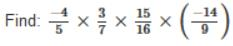Solution:

-4/5 ×3/7×15/16×(-14/9) =-4/5 × -2/9 ×.15/16 = 1/2

QUESTION: 9

Which of the following is the product of 7/8 and -4/21?

Solution:

According to question, 7/8 * -4/21 = -1/6

QUESTION: 10

Compare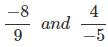Solution:

Expressing in standard form, we get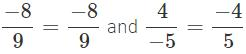Express each rational number with L.C.M as denominator. L.C.M of 9 and 5 is 9×5 = 45.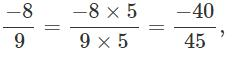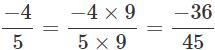Comparing the numerators, we have

−40 <−36.

∴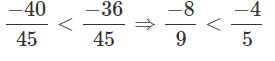QUESTION: 11

_____ is the only rational number that is equal to its reciprocal.

Solution:

1 because the reciprocal of 1 is 1/1 which is equal to 1.

QUESTION: 12

________ are closed under subtraction.

Solution:

Integers: This set is closed only under addition, subtraction, and multiplication. Rational Numbers: This set is closed under addition, subtraction, multiplication, and division (with the exception of division by 0). Irrational Numbers: This set is closed for none of the operations

QUESTION: 13

Zero has ________ reciprocal.

Solution:

Zero has no reciprocal. Because 1/0 is not defined and also remember any number multiplied by zero gives 0. Therefore, if reciprocal was supposed to be there then that reciprocal when multiplied by 0 should give 1 which is not possible.

QUESTION: 14

The product of two rational numbers is always a _______.

Solution:

Product of two rational numbers is always a rational number.
For example,
1/2 + 1/2 = 1

QUESTION: 15

A number which can be written in the form, p/q where p and q are integers and _____ is called a rational number.

Solution:

If q = 0, the number would become undefined.
Therefore, q ≠ 0 is the answer.

QUESTION: 16

Find the multiplicative inverse of 2/9.

Solution:

Multiplicative inverse is nothing but a reciprocal of a number 2/9 which is 9/2.

QUESTION: 17

The product of two numbers is -20/9. If one of the numbers is 4, find the other.

Solution:

Let the other number be R
One number = 4
Given,
4*R = -20/9
Therefore, R = (-20/9)/4
= -5/9

QUESTION: 18

Find a rational number between 1/4 and 1/2.

Solution:

a = 1/4, b = 1/2
Therefore, rational no. b/w 1/4 and 1/2 is
(1/4 + 1/2)/2 = 3/8

QUESTION: 19

1 is the __________ for rational numbers.

Solution:

If you multiply any number with 1,the product will always be the same number which you multiply with 1. Therefore, 1 is the multiplicative identity.

QUESTION: 20